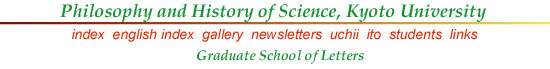### Famous Logicians and Philosophers of Mathematics by S. UchiiG. Cantor (1845-1918): Founder of the Set Theory, Inquiry into the Infinity. "There are more real numbers than natural numbers";"Is there any infinity which is larger than the denumerable and smaller than the continuum?"G. Frege (1848-1925): Inventor of the Mathematical Logic, Logicist Foundation of Arithmetic. "A proposition is made of a function and arguments"; "Mathematics can be reduced to logic."B. Russell (1872-1970): Russell Paradox, Logicist Philosopher of Mathematics. "Is the present King of France bald?"; "Consider the set of all sets which do not belong to themselves; does this set belong to itself?"D. Hilbert (1862-1943): Formalist Philosopher of Mathematics, Hilbert's program. "We should be able to say that axioms may be satisfied by a table, a chair, or a mug"; "If we show consistency within Finitism, classical mathematics can be saved."L.E.J. Brower (1881-1966): Intuitionist Philosopher of Mathematics. "What is not constructive is not mathematics"; Proof is more fundamental than truth.J. von Neumann (1903-1957): Automata Theory, Design of Computer. "Five tubes for logic, five tubes for electronics"; his calculation was faster than computers!K. Goedel (1906-1978): Highlights of mathematical logic and axiomatic set theory. "Any consistent axiomatization of arithmetic is incomplete; i.e., there is an arithmetical truth such that neither itself nor its negation can be proved in it."A.M. Turing (1912-1954): "Computable means what can be done by a Turing machine"; "If a machine passes certain tests, it may be regarded as intelligent and capable of thinking."